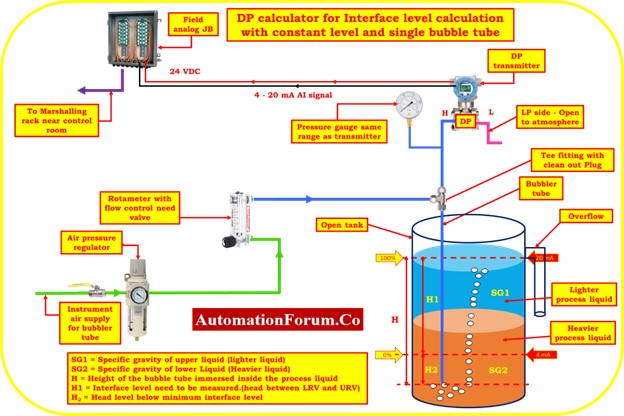# DP calculator for bubbler method Interface level measurement – Transmitter installed with Constant Tank Level and single Bubble TubeURV = Upper-range value (a measurement with a 20 mA output).

SG = A liquid’s specific gravity (relative density). Water has a specific gravity of 1.00 in both traditional and SI systems.

HW= Equivalent Headlevel pressure of Water

SG1 = Specific gravity of upper liquid (lighter liquid)

SG2 = Specific gravity of lower Liquid (Heavier liquid)

H = Height of the bubble tube immersed inside the process liquid

H1 = Interface level need to be measured.(head between LRV and URV)

H2 = Head level below minimum interface level

## Formula for Span value calculation

Span value of the transmitter  =  (H1)*(SG2 – SG1)

## Formula for LRV estimation

Equivalent Head level pressure of Water(HW) at Lower range value

HW at LRV = (H1*SG1) + (H2*SG2) = Suppression

## Formula for URV estimation

Equivalent Head level pressure of Water(HW) at Higher range value

Hw at URV =  (H1 +H2)*SG2

## DP calculator

This below calculator used to calculate the Span value, LRV and URV of the transmitter for calibration in interface level measurement